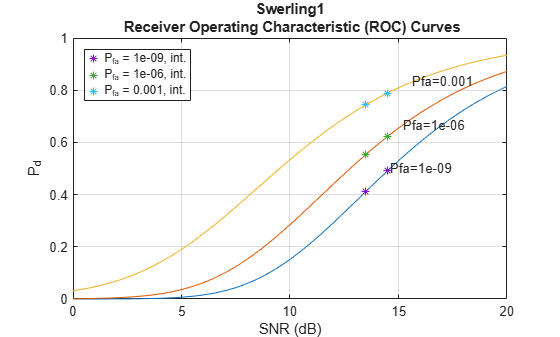# rocinterp

ROC curve interpolation

## Syntax

``ipd = rocinterp(snr,pd,snrq,'snr-pd')``
``isnr = rocinterp(pd,snr,pdq,'pd-snr')``
``ipd = rocinterp(pfa,pd,pfaq,'pfa-pd')``
``ipfa = rocinterp(pd,pfa,pdq,'pd-pfa')``

## Description

example

````ipd = rocinterp(snr,pd,snrq,'snr-pd')` returns the probability of detection (Pd) computed by interpolating a Pd vs. signal-to-noise ratio (SNR) receiver operating characteristic (ROC) curve. If `pd` is a matrix, the function interpolates each column independently. In this and the next syntax, `rocinterp` performs linear interpolation after transforming the Pd-axis of the ROC curve using the normal probability scale.```

example

````isnr = rocinterp(pd,snr,pdq,'pd-snr')` returns the SNR computed by interpolating a Pd vs. SNR ROC curve. If `snr` is a matrix, the function interpolates each column independently.```

example

````ipd = rocinterp(pfa,pd,pfaq,'pfa-pd')` returns the Pd computed by interpolating a Pd vs. probability of false alarm (Pfa) ROC curve. If `pd` is a matrix, the function interpolates each column independently. In this and the next syntax, `rocinterp` performs linear interpolation after transforming both axes of the ROC curve using a logarithmic scale.```

example

````ipfa = rocinterp(pd,pfa,pdq,'pd-pfa')` returns the Pfa computed by interpolating a Pd vs. Pfa ROC curve. If `pfa` is a matrix, the function interpolates each column independently.```

## Examples

collapse all

Compute the probability of detection (${\mathit{P}}_{\mathrm{d}}$) for a Swerling 1 case target given a set of signal-to-noise ratio (SNR) and probability of false alarm values. Express the SNR values in decibels.

```SNR = [13.5 14.5]; pfa = [1e-9 1e-6 1e-3];```

Compute the ${\mathit{P}}_{\mathrm{d}}$ vs. SNR ROC curves and interpolate them at the SNR values of interest.

```[pd,snr] = rocpfa(pfa,'SignalType','Swerling1'); ipd = rocinterp(snr,pd,SNR,'snr-pd');```

Plot the ROC curves and overlay the interpolated values.

```rocpfa(pfa,'SignalType','Swerling1') hold on q = plot(SNR,ipd,'*'); hold off legend(q,append("P_{fa} = ",string(pfa),", int."),'Location','northwest')```## Input Arguments

collapse all

Signal-to-noise ratio in decibels (dB), specified as a vector or matrix. If `snr` is a vector, its values must be unique. If `snr` is a matrix, then each of its columns must contain unique values.

Data Types: `double`

Signal-to-noise ratio query points, specified as a vector. All values of `snrq` must be expressed in dB.

Data Types: `double`

Probability of detection, specified as a vector or matrix. All values of `pd` must be between 0 and 1. If `pd` is a vector, its values must be unique. If `pd` is a matrix, then each of its columns must contain unique values.

Data Types: `double`

Probability of detection query points, specified as a vector. All values of `pdq` must be between 0 and 1.

Data Types: `double`

Probability of false alarm, specified as a vector or matrix. All values of `pfa` must be between 0 and 1. If `pfa` is a vector, its values must be unique. If `pfa` is a matrix, then each of its columns must contain unique values.

Data Types: `double`

Probability of false alarm query points, specified as a vector. All values of `pfaq` must be between 0 and 1.

Data Types: `double`

## Output Arguments

collapse all

Interpolated probability of detection, returned as a vector or matrix.

Interpolated signal-to-noise ratio, returned as a vector or matrix.

Interpolated probability of false alarm, returned as a vector or matrix.

## Extended Capabilities

### C/C++ Code GenerationGenerate C and C++ code using MATLAB® Coder™.